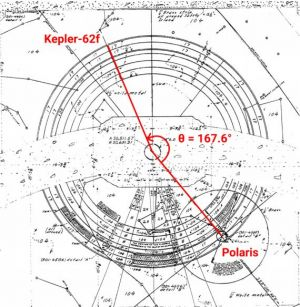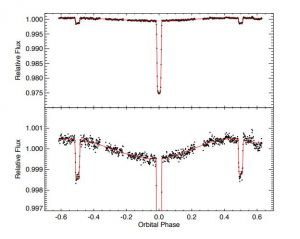# USAAAO2019

## 相关链接

#### 英文题目

The maximum score is 153 points. The test must be completed within 2.5 hours (150 minutes).

##### 短问题

1. (7 points) Assuming that the present density of baryonic matter is ρb0 = 4.17*10^28 kg/m3 , what was the density of baryonic matter at the time of Big Bang nucelosynthesis (when T = 10^10 K)? Assume the present temperature, T0 to be 2.7 K.

2. (7 points) On the night of January 21st, 2019, there was a total lunar eclipse during a supermoon. At the time, the moon was close to perigee, at a distance of 351837 km from the earth, which was 1.4721*10^8 km from the sun. The gamma (γ) of a lunar eclipse refers to the closest distance between the center of the moon and the center of the shadow, expressed as a fraction of the earth’s radius. For this eclipse, γ = 0.3684. Given this information, find the closest estimate for the duration of totality of the eclipse.

3. (7 points) You are in the northern hemisphere and are observing rise of star A with declination δ = -8 degrees , and at the same time a star B with declination δ = +16 degrees is setting. What will happen first: next setting of the star A or rising of the star B?

4. (7 points) Consider a star with mass M and radius R. The star’s density varies as a function of radius r according to the equation ρ(r) = ρ(center)(1-sqrt(r/R)), where ρcenter is the density at the center of the star. Derive an expression for dP{dr in terms of G, M, R, and r, where P is the pressure at a given radius r.

##### 中问题

5. (15 points) An alien spaceship from the planet Kepler 62f is in search of a rocky planet for a remote base. They’re attracted to Earth because of a fortunate coincidence: its axis of rotation points directly at their home planet. That means they can have uninterrupted communication with home by planting fixed transmitters on The North Pole. But first, they need to find out if Earth’s axis will always point in the same direction or if it undergoes precession. They can’t know without years of observation, so they hope that we, its now-extinct intelligence, have left behind the answer. While orbiting Earth, they see a few remarkable structures, including the Hoover Dam in Nevada. Zooming in on the dam, a colorful plaza with peculiar markings on its floor catches their attention. Descending on the plaza, they realize the markings are a map of the sky when the dam was built, left to indicate the date to posterity. Figure 1 is an overhead architectural map of this plaza. The center-point depicts the north ecliptic pole, and the large circle represents the path of the Earth’s axis throughout its counter-clockwise procession. As they interpret the map, they’re dismayed to realize that their star has not been and will not be Earth’s north star for very long.Figure 1: Overhead architectural plan of the Hoover Dam plaza depicting Polaris as north star

For the purpose of this question, assume that the Earth’s axial tilt is a constant i = 23.5 degrees and its axis precesses at a constant rate.

a) Using the values on the map, and knowing that the aliens used carbon-aging to determine that the dam is 12,000 years old, find all possible values for the period of Earth’s axial precession.

b) Using the most optimistic answer (longest period) from part (a), calculate how many arcseconds the Earth’s axis precesses each day. Use the period you calculate here in the next two sections.

c) If they hadn’t been lucky enough to come across the star map and decided to build a radio interferometer to observe the movement of the celestial pole over the course of 30 days instead, how many kilometers would the baseline of their telescope array have to be, assuming it operated at a 20cm wavelength?

d) As a last resort, to keep Earth’s axis fixed, the aliens decide to counter the forces that cause the Earth’s precession by building giant nuclear thrusters on the Earth’s surface. Assume Earth’s precession is caused by external forces alone and calculate the average force (in kN) that a strategically positioned thruster would have to exert to counter them.

6. (15 points)Figure 2 shows a full-phase light curve (“phase curve”) of the exoplanet HD 189733b taken by the Spitzer space telescope.

Use this figure to answer the following questions.

The star HD 189733 has an effective temperature of 4785 K and a radius of 0.805 Solar radii.

a) Use the depth of the planet’s transit to estimate the radius of HD 189733b, in Jupiter radii.

b) Use the depth of the eclipse of the planet by the host star to estimate the ratio of the flux of the planet HD 189733b to that of the host star HD 189733.

c) HD 189733b is so close-in to its host star that it is expected to be tidally locked. Use the phase curve to estimate the ratio of the dayside flux emitted by the planet to the nightside flux emitted by the planet.

d) This phase curve also noticeably has a phase curve offset, that is, the maximum in planet and star flux does not occur exactly at secondary eclipse. What process that occurs in a planetary atmosphere could cause such a phase curve offset?

7. (15 points)

a) Mass-Radius Relation Stellar physics often involves guessing the equation of state for stars, which is typically a relation between the pressure P and the density ρ. A family of such guesses are known as polytopes and go as follows

P = K*ρ^γ (1)

where K is a constant and the exponent γ is fixed to match a certain pressure and core temperature of a star. Given this, show that one can obtain a crude power-law scaling between the mass M of a polytopic star and its radius R of the form M9Rα. Find the exponent α for polytopic stars (justify all steps in your argument). Also, indicate the exponent γ for which the mass is independent of the radius R. Bonus: Why is this case interesting?

b) Black Holes as Blackbodies The mass radius relation for ideal non-rotating, uncharged black holes is known from relativity to be

R = 2GM/c^2 (2)

Moreover, Stephen Hawking showed that a black hole behaves like a blackbody, where its temperature (known as the Hawking temperature) is given by

T = ħc^3/8πkGM (3)

Given this information, show that the lifetime of a black hole (justify this phrase!) t ˚ scales with its mass M as

t* is proportional to Mβ (4)

where you should find the exponent β

c) Minimal Black Holes Using the information of the previous part, and Wien’s displacement law, estimate the smallest possible mass of a black hole. State any possible flaws with this estimate.

8. (15 points) In a rather weird universe, the gravitational constant G varies as a function of the scale factor aptq. G “ G0fpaq (5) Consider the model fpaq “ e bpa´1q where b “ 2.09. a) Assuming that the universe is flat, dark energy is absent, and the only constituent is matter, estimate the present age of this weird universe according to this model. Assume that the Friedmann equation: Hpaq 2 “ H2 0 pΩm ` Ωr ` Ωk ` ΩΛq (6) still holds in this setting. b) What is the behaviour of the age of the universe t as the scale factor aptq Ñ 8 ? Note that all parameters with subscript 0 indicate their present value. Take the value of Hubble’s constant as H0 “ 67.8 kms´1Mpc´1 Hint: You might need the following integrals ż 8 0 x 2 e ´x 2 dx “ ? π 4 ż 1 0 x 2 e ´x 2 dx « 0.189471 (7) 9. (15 points) a) Find the shortest distance from Boston (42.36010 N, 71.05890 W) to Beijing (39.90420 N, 116.40740 E)traveling along the Earth’s surface. Assume that the Earth is a uniform sphere of radius 6371 km. b) What fraction of the path lies within the Arctic circle (north of 66.56080 N)?